# Heisenberg Uncertainty Principle: Equation & Explanation

Contents

## What is the Heisenberg Uncertainty Principle?

The Heisenberg Uncertainty Principle is one of the more interesting and consequential outcomes of the statistical nature of quantum mechanics. The most famous realization of the uncertainty principle states that one cannot measure with absolute certainty the position and momentum of a quantum system. This is the most common realization that is found all over popular science. However, the uncertainty principle is much more generalized than this. The full uncertainty principle is also characterized by inequality. The generalized uncertainty principle is expressed as:

Only a special case of this generalized relationship applies to position and momentum which attracts the most attention. In this article, we will explore what it means to measure something, the uncertainty principle, and finally, the Heisenberg uncertainty principle (they’re not the same thing!).

The Heisenberg uncertainty principle is a consequence of the statistical nature of quantum mechanics. In order to understand it, one must first understand measurement.

## What is Measurement in Quantum Physics?

The act of measurement disturbs a quantum system. For example, if you were to perform any measurements like in the article for electrical measurements you would disturb the underlying electrical system of particles (you would also quickly find that you cannot ever measure with absolute certainty – but that is to be covered at a later date).

The disturbance on the quantum system is because the process of measurement is actually the same as acting on a wave function of the quantum system (which satisfies the Schrodinger Equation) with an operator.

Once the operator acts on the wave function, the wave function will collapse into an eigenstate corresponding to that operator. What a mouthful! Let’s take a step back from all of this jargon and go back to first principles.

Let’s conduct a thought experiment about the photoelectric effect by trying to measure where exactly on the other end of the slit the particle will land (imagine you have a ruler and you’re trying to measure where along the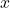axis the particle will land). One of the main results from early-stage quantum physics was that particles behave like waves.

That means that there is a non-zero probability of finding the particle anywhere along theaxis. Let’s look at a few probability distributions around the measurement of position. If we had a semi-certain idea of where the particle is located, the error (or the standard deviation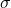) of the position measurement’s distribution would look something like:

If we somehow knew exactly where the particle was located along the position axis, the probability distribution would look a little something like:

If we had little to no idea where the particle was located on the other end of the slit, the probability distribution would look something like:

This is where the statistical nature of quantum mechanics comes into play. Qualitatively, the wave function provides a probability distribution for each observable we want to measure (think energy, momentum, position, total energy, etc).

## Deriving the Heisenberg Uncertainty Principle

The derivation of the generalized uncertainty formula will be covered at a later stage. The first theoretical milestone has to do with understanding that every time a measurement is to be made, an operator acts on the state vector representing the quantum system. For position, the operator is given as (where the hat implies that it’s an operator):

So when you measure the position of a quantum system, you’re multiplying the state by. For momentum, the operator looks like:

This is where the conversation around incompatible observables comes to play. Any time the operators associated with the observables have a non-zero commutator, you cannot observe both with certainty. The commutator for two operators is defined as follows:

This commutator of the two operators corresponding to the observables we are trying to measure is upheld by the generalized uncertainty principle formula. Let us now compute the commutator for position and momentum:

Therefore if you substitute this result into the generalized uncertainty principle formula, you obtain the famous Heisenberg uncertainty principle: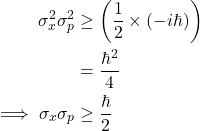This is the famous realization of the uncertainty principle. It can be interpreted as follows: as you become more and more certain about the location of your quantum system (i.e.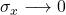, the more uncertain you will become about the momentum of the system (i.e.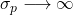and vice-versa.

Again, this is due to the fact that the standard deviation will blow up for the momentum as the standard deviation for position becomes smaller (more certainty) to uphold the uncertainty principle.

## Citations

Want To Learn Faster? 🎓
Get electrical articles delivered to your inbox every week.
No credit card required—it’s 100% free.